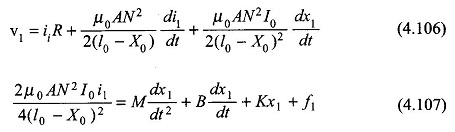## Dynamical Equations of Electromechanical Systems:

Dynamical Equations of Electromechanical Systems – Figure 4.19 shows an electromagnetic relay whose armature is loaded with spring K, damper B, mass M and a force generator F.

Figure 4.20 shows the abstracted diagram of a general electromechanical systems. It is easily noticed that the electromechanical device has one electrical port and one mechanical port (one terminal of the mechanical port being the ground) through which it is connected to the electrical source on one side and mechanical load on the other side. In general there can be more than one electrical port (multiply excited system).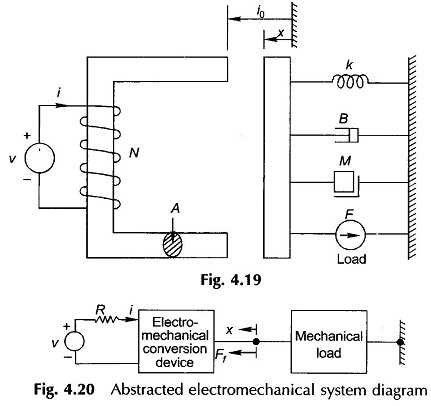Let the electromechanical device has an inductance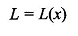The governing electrical equation is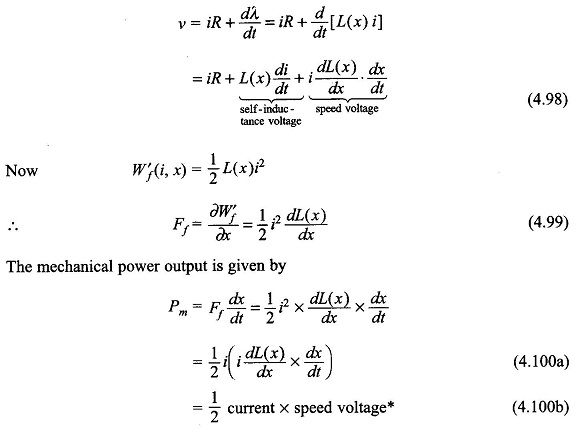Mechanical power output results (i.e. electrical power is converted to mechanical form) when the current in the device flows in opposition to the speed voltage. When the current in the device is in the same direction as the speed voltage, electrical power is output, i.e., mechanical power is converted to electrical form.

The governing differential equation of the mechanical system is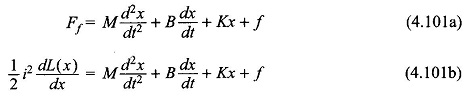Now for the specific system of Fig. 4.18, when the armature is in position x, the self-inductance L is found below: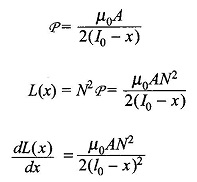Substituting in Eqs. (4.98) and (4.99), the two differential equations defining the system’s dynamic behaviour are obtained as: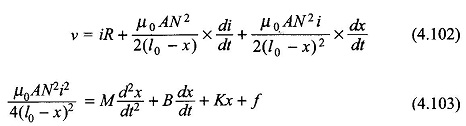These are nonlinear differential equations which can be solved numerically on the digital computer. However, for small movement around the equilibrium point the following procedure can be adopted.

Let the equilibrium point be (V0,I0,X0,f0). At equilibrium the system is stationary and all derivatives are zero. Thus from Eqs (4.102) and (4.103), the following relationships between equilibrium values are obtained.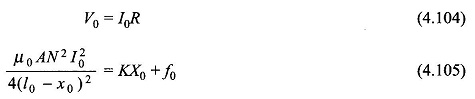Let the departure (small) from the equilibrium values be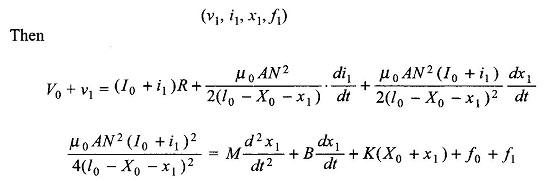Neglecting products of small departures and small departures compared to equilibrium values, and also cancelling out equilibrium terms as per Eqs (4.104) and (4.105),